## 东风风行 风行CM7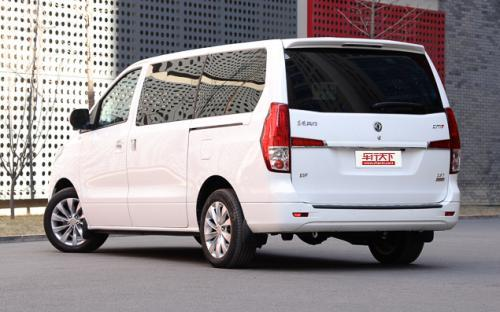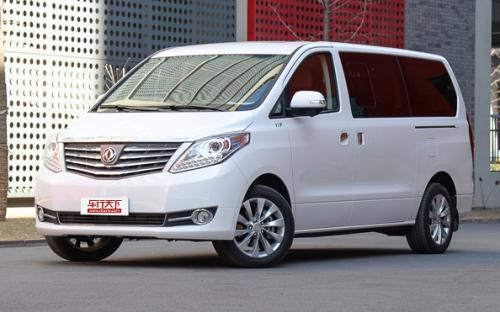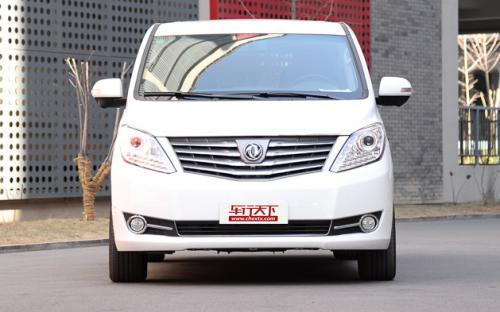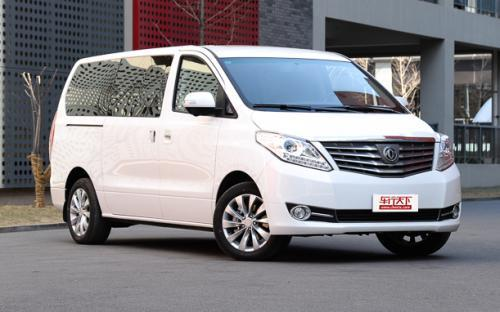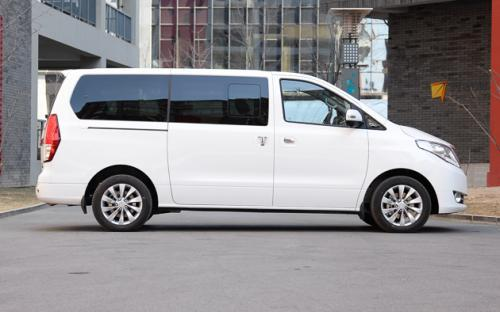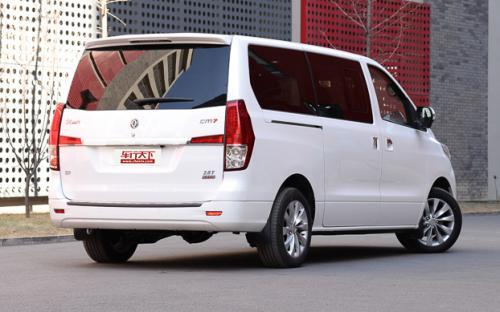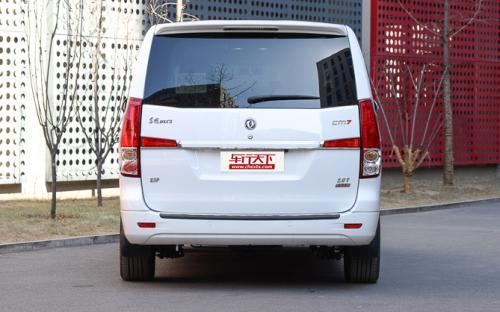2 种颜色可选2016款最低售价：14.99 万元起

5150(mm)1920(mm)1925(mm)##### 配置亮点：
• 胎压监测装置

• ISOFIX儿童座椅接口

• 车身稳定控制(ESC/ESP/DSC等)

• 电动天窗

• 定速巡航

• 后倒车雷达

• 真皮座椅

• GPS导航系统

• 氙气大灯

• 后视镜加热

2016款 2.0T 自动尊享型 (221张)
• 2016款 2.0T 自动尊享型 (221张)
• 2016款 2.0T 自动尊贵型 (151张)
• 2016款 2.0T 自动旗舰型 (35张)
• 2016款 2.0T 手动尊享型 (145张)东风风行 风行CM7 在售车型

排量 车型 厂商指导价 本地最低报价 购车工具
2.0T 2.0T 手动豪华型 6挡手动 14.99万 14.99万

询底价+对比
2.0T 手动尊享型 6挡手动 15.99万 询底价+对比
2.0T 自动豪华型 6挡自动 16.69万 16.69万

询底价+对比
2.0T 自动尊享型 6挡自动 17.99万 询底价+对比
2.0T 自动尊贵型 6挡自动 19.99万 询底价+对比
2.0T 自动旗舰型 6挡自动 21.99万 21.99万

询底价+对比

东风风行 风行CM7 经销商

查看更多 >>
更多 >>

本地降价排名

### 东风风行 风行CM7 动力加速

风行CM7 0-100公里加速时间分布在 0.0-秒 属于 超跑级

动力级别 加速时间 车型

东风风行 风行CM7 视频

东风风行 风行CM7 新闻资讯

# 2015广州车展 新款东风CM7售14.99万起

国产新车 超过8335次关注

2015广州车展上，东风风行推出了2016款CM7车型，在外形和内饰设计上与手动挡车型保持一致，2亮点是配备了2.0T+6AT的动力组合，新车全系的售价区间为14.99-21.99万元。

# 静态体验东风风行CM7 外形上档次

导购 超过3550次关注

东风风行目前在售的MPV仅菱智一款，虽然销量在同级中表现出色，但面对竞争对手丰富的产品线难免显得势单力薄，伴随商务车需求的高端化，风行CM7便应运而生，该车定...

# 空间宽敞/配置丰富 实拍东风风行CM7

导购 超过3473次关注

2013上海车展上，东风风行发布了旗下最新的高端商务MPV车型风行CM7，与此前该品牌下的风行菱智相比，风行CM7不仅外观内饰更显档次，配置也更加丰富。而在同级别车型...

# 东风风行CM7实拍 向着更高的市场进发

导购 超过3207次关注

对于国内的商务MPV车型市场来说，东风风行目前除了菱智外，暂时没有一款更为偏重商务的车型能够与金杯阁瑞斯、福田蒙派克等车型竞争。不过，在2013上海车展我们将会...

# 2014成都车展探馆：东风风行CM7 2.0T

新闻 超过2273次关注

2014成都车展即将于8月29日开幕，日前，我们的编辑在探馆时提前拍到了东风风行CM72.0T车型的图片，这款车将会在车展上正式上市。

# 成都车展上市 东风风行推CM7 2.0T车型

新闻 超过2425次关注

近日我们从官方渠道获悉，东风风行将会在成都车展期间公布CM72.0T车型的市场指导价格，加上此前上市的2.4L车型，CM7将会提供更加丰富的车款选择。

# 东风风行CM74于车展前上市 预售13-20万元

新闻 超过2398次关注

东风风行CM7定位于中高端商务MPV。外观方面，新车采用传统商务MPV造型，前脸进气格栅采用镀铬装饰的四幅条设计，配合不规则的前大灯组使新车看起来非常大气稳重。

猜你喜欢

﻿
• 快速找车
• 选择品牌
• 选择品牌
• A  奥迪
• A  阿斯顿·马丁
• A  阿尔法·罗密欧
• B  宝沃
• B  布加迪
• B  巴博斯
• B  保时捷
• B  宾利
• B  奔驰
• B  宝马
• B  本田
• B  别克
• B  标致
• B  比亚迪
• B  宝骏
• B  北汽制造
• B  北汽新能源
• B  北汽幻速
• B  北汽威旺
• B  北京汽车
• B  奔腾
• B  北汽绅宝
• C  长安
• C  长安商用
• C  长城
• C  昌河
• D  大众
• D  道奇
• D  DS
• D  东南
• D  东风风神
• D  东风风行
• D  东风小康
• D  东风风度
• D  东风
• F  福特
• F  丰田
• F  菲亚特
• F  法拉利
• F  福田
• F  福迪
• F  福汽启腾
• G  观致
• G  广汽传祺
• G  广汽吉奥
• G  GMC
• H  红旗
• H  汉腾汽车
• H  哈弗
• H  哈飞
• H  海格
• H  海马
• H  华颂
• H  黄海
• H  华泰
• H  恒天
• J  吉利汽车
• J  捷豹
• J  Jeep
• J  江淮
• J  江铃
• J  金杯
• J  九龙
• J  金旅
• K  凯翼
• K  凯迪拉克
• K  克莱斯勒
• K  科尼塞克
• K  卡威
• K  开瑞
• L  路虎
• L  林肯
• L  劳斯莱斯
• L  兰博基尼
• L  雷克萨斯
• L  铃木
• L  雷诺
• L  理念
• L  力帆
• L  莲花汽车
• L  猎豹
• L  路特斯
• L  陆风
• M  马自达
• M  MG
• M  MINI
• M  玛莎拉蒂
• M  摩根
• M  迈凯轮
• N  纳智捷
• O  欧宝
• O  讴歌
• O  欧朗
• Q  奇瑞
• Q  起亚
• Q  启辰
• R  日产
• R  荣威
• R  瑞麒
• S  三菱
• S  斯威汽车
• S  萨博
• S  smart
• S  斯柯达
• S  斯巴鲁
• S  思铭
• S  双龙
• S  上汽大通
• S  双环
• T  特斯拉
• T  腾势
• W  沃尔沃
• W  五菱汽车
• W  五十铃
• W  威兹曼
• W  威麟
• X  现代
• X  雪佛兰
• X  雪铁龙
• X  西雅特
• Y  一汽
• Y  英菲尼迪
• Y  英致
• Y  依维柯
• Y  野马汽车
• Y  永源
• Z  众泰
• Z  中华
• Z  中兴
• Z  知豆
• 选择车系
• 选择车系
• 车型对比
• 选择品牌
• 选择品牌
• A  奥迪
• A  阿斯顿·马丁
• A  阿尔法·罗密欧
• B  宝沃
• B  布加迪
• B  巴博斯
• B  保时捷
• B  宾利
• B  奔驰
• B  宝马
• B  本田
• B  别克
• B  标致
• B  比亚迪
• B  宝骏
• B  北汽制造
• B  北汽新能源
• B  北汽幻速
• B  北汽威旺
• B  北京汽车
• B  奔腾
• B  北汽绅宝
• C  长安
• C  长安商用
• C  长城
• C  昌河
• D  大众
• D  道奇
• D  DS
• D  东南
• D  东风风神
• D  东风风行
• D  东风小康
• D  东风风度
• D  东风
• F  福特
• F  丰田
• F  菲亚特
• F  法拉利
• F  福田
• F  福迪
• F  福汽启腾
• G  观致
• G  广汽传祺
• G  广汽吉奥
• G  GMC
• H  红旗
• H  汉腾汽车
• H  哈弗
• H  哈飞
• H  海格
• H  海马
• H  华颂
• H  黄海
• H  华泰
• H  恒天
• J  吉利汽车
• J  捷豹
• J  Jeep
• J  江淮
• J  江铃
• J  金杯
• J  九龙
• J  金旅
• K  凯翼
• K  凯迪拉克
• K  克莱斯勒
• K  科尼塞克
• K  卡威
• K  开瑞
• L  路虎
• L  林肯
• L  劳斯莱斯
• L  兰博基尼
• L  雷克萨斯
• L  铃木
• L  雷诺
• L  理念
• L  力帆
• L  莲花汽车
• L  猎豹
• L  路特斯
• L  陆风
• M  马自达
• M  MG
• M  MINI
• M  玛莎拉蒂
• M  摩根
• M  迈凯轮
• N  纳智捷
• O  欧宝
• O  讴歌
• O  欧朗
• Q  奇瑞
• Q  起亚
• Q  启辰
• R  日产
• R  荣威
• R  瑞麒
• S  三菱
• S  斯威汽车
• S  萨博
• S  smart
• S  斯柯达
• S  斯巴鲁
• S  思铭
• S  双龙
• S  上汽大通
• S  双环
• T  特斯拉
• T  腾势
• W  沃尔沃
• W  五菱汽车
• W  五十铃
• W  威兹曼
• W  威麟
• X  现代
• X  雪佛兰
• X  雪铁龙
• X  西雅特
• Y  一汽
• Y  英菲尼迪
• Y  英致
• Y  依维柯
• Y  野马汽车
• Y  永源
• Z  众泰
• Z  中华
• Z  中兴
• Z  知豆
• 选择车系
• 选择车系
• 选择车型
• 选择车型
• 意见反馈